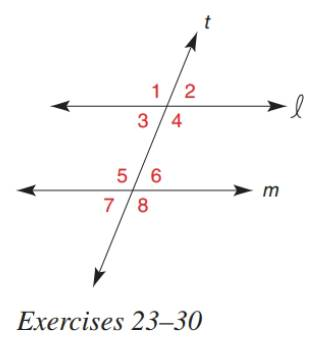Chapter 2.3, Problem 23E### Elementary Geometry for College St...

6th Edition
Daniel C. Alexander + 1 other
ISBN: 9781285195698

#### Solutions

Chapter
Section### Elementary Geometry for College St...

6th Edition
Daniel C. Alexander + 1 other
ISBN: 9781285195698
Textbook Problem
3 views

# In Exercise 23 to 30, determine the value of x so that line l will be parallel to line m . m ∠ 4 = 5 x m ∠ 5 = 4 ( x + 5 )To determine

To find:

The value of x for the given statement.

Explanation

Given:

The given statement is,

Line 𝓁 and m are cut by transversal t and are parallel to each other.

m4=5x and m5=4(x+5).

Figure (1)

Theorem:

If two parallel lines are cut by transversal, then the pairs of alternate interior angles are congruent.

Calculation:

Line 𝓁 is parallel to line m.

4 and 5 are alternate interior angles.

So,

### Still sussing out bartleby?

Check out a sample textbook solution.

See a sample solution

#### The Solution to Your Study Problems

Bartleby provides explanations to thousands of textbook problems written by our experts, many with advanced degrees!

Get Started

#### In Problems 23-28, graph the functions.

Mathematical Applications for the Management, Life, and Social Sciences

#### Explain what is meant by the statement limx2f(x)=3.

Applied Calculus for the Managerial, Life, and Social Sciences: A Brief Approach

#### Convert the expressions in Exercises 8596 radical form. 22/3

Finite Mathematics and Applied Calculus (MindTap Course List)

#### Express the function in the form f g h. 51. S(t) = sin2(cos t)

Single Variable Calculus: Early Transcendentals, Volume I

#### Find the median for the following set of scores: 1, 9, 3, 6, 4, 3, 11, 10

Essentials of Statistics for The Behavioral Sciences (MindTap Course List)

#### What is the integrating factor for xy′ + 6x2y = 10 − x3?

Study Guide for Stewart's Single Variable Calculus: Early Transcendentals, 8th